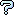All about flooble | fun stuff | Get a free chatterbox | Free JavaScript | Avatarsperplexus dot infoLetter Cubes 3 (Posted on 2003-08-17)In this game of Letter Cubes, a different letter of the alphabet is on each face of each of the 4 cubes so that 24 of the 26 letters of the alphabet occur. Words are formed by rearranging and turning the cubes so that the top letters spell a common 4-letter word. The 14 words below have been made using today's cubes.

Can you recover the 6 letters on each?
```AXIS
BUOY
FAKE
FOIL
GROW
HOAR
JUMP
MUCK
NAVY
OPTS
TURN
VIBE
```

(puzzle originally from www.allstarpuzzles.com)

 Submitted by DJ Rating: 4.2000 (15 votes) Solution: (Hide) ``` A B L M T W C E J N O X D I K P R Y F G H S U V ``` According to the introduction, each of the 24 faces of the dice has a different letter on it. Given the word GOAD, we arbitrarily assign G to die 1, O to die 2, A to die 3, and D to die 4. ```G O A D ``` From GROW, R can't go on a die with G or O; and from HOAR, R isn't on die 3 with A. R is on die 4 with D. ```G O A D R ``` From GROW, then, the W is on die 3 with A; and from HOAR, H is on the same die as G. ```G H O A W D R ``` From CHAD, C is on die 2 with O. ```G H O C A W D R ``` F isn't on die 2 with O (FOIL) or die 3 with A (FAKE), so F is on die 1 or die 4. Start by assuming that F is on cube 4.If F were with DR, given that I isn't on the same cube as A (AXIS), from FOIL, I would go with GH and L with AW. ```G H (I) O C A W (L) D R (F) ``` Since K doesn't go with C on a die (MUCK), from FAKE we would get GHIK on die 1 and OCE on die 2. ```G H (IK) O C (E) A W (L) D R (F) ``` Given SPOT, S can't be on a die with O; therefore, given AXIS, die 2 would have X and die 4 S. ```G H (IK) O C (EX) A W (L) D R (FS) ``` V isn't on the same die as A (NAVY); so, given VIBE, V would be on the fifth face of die 4 and B on die 3. ```G H (IK) O C (EX) A W (LB) D R (FSV) ``` Y doesn't occupy the same die as V (NAVY), so Y in BOUY would go on die 1. ```G H (IKY) O C (EX) A W (LB) D R (FSV) ``` Then, however, the U cannot be on die 1, 2, 3 (BUOY), or 4 (TURN). Therefore the assumption that F is on cube 4 is false; F is on die 1. ```G H F O C A W D R ``` I isn't on with A (AXIS), so from FOIL, I is on die 4 and L on die 3. ```G H F O C A W L D R I ``` Given MUCK, K and C are on different blocks; from FAKE, then, the K goes with DRI and the E with OC. ```G H F O C E A W L D R I K ``` S isn't on a die with O (SPOT); so, from AXIS, S is on die 1 with GHF and X on the second cube. ```G H F S O C E X A W L D R I K ``` The T is not on die 1, 2 (SPOT), or 4 (TURN); it is on 3, and the P in SPOT is on die 4. ```G H F S O C E X A W L T D R I K P ``` U isn't on with O (BUOY); so given TURN, the U is on cube 1 with GHFS and the N with OCEX. ```G H F S U O C E X N A W L T D R I K P ``` Using VIBE, V isn't on with A (NAVY) and completes block 1; B is on die 3 with AWLT. ```G H F S U V O C E X N A W L T B D R I K P ``` Y finishes cube 4 (NAVY), M is on cube 3 (MUCK), and J is on a face of 2 (JUMP). ```G H F S U V O C E X N J A W L T B M D R I K P Y ``` Then, for aesthetics, rearrange the cubes and letters by alphabetical order: ```A B L M T W C E J N O X D I K P R Y F G H S U V ``` Charlie posed an interesting method using a grid to do some of the elimination, that can be found here.Subject Author Datere(3): please ignore SilverKnight 2003-10-10 13:23:30re(2): please ignore FatBoy 2003-10-10 12:35:31re: please ignore SilverKnight 2003-10-10 11:14:07please ignore FatBoy 2003-10-10 10:15:20please ignore FatBoy 2003-10-10 10:15:12No Subject Khanh 2003-08-26 17:40:38Spreadsheet helps Lawrence 2003-08-23 07:35:59Solution Shelly 2003-08-21 22:26:53Solution Donna 2003-08-21 14:19:17solution Jonathan Shaw 2003-08-19 03:15:43re: solution DuCk 2003-08-18 12:27:44re: solution kyle 2003-08-18 02:15:26solution Charlie 2003-08-17 11:16:21Please log in:

 Search: Search body:
Forums (1)